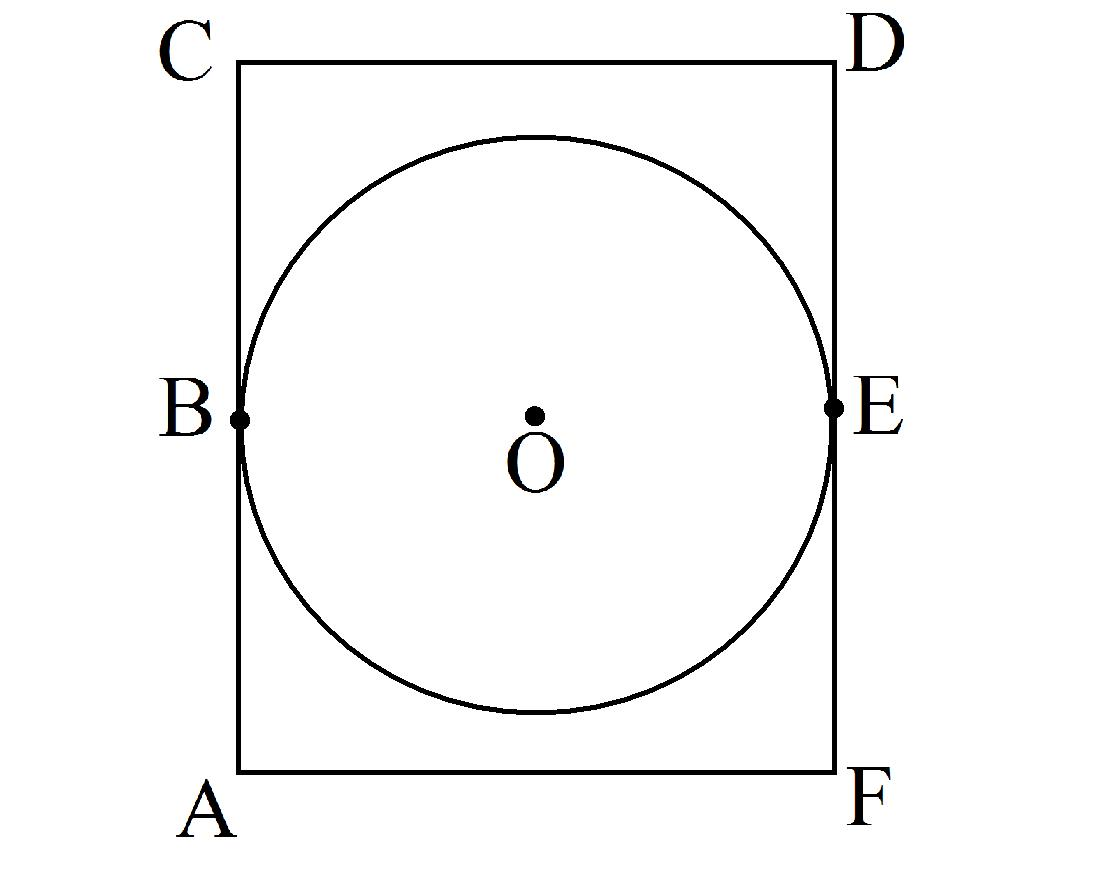# SAT Math IX

Geometry Level 2In the figure above, $ACDF$ is a rectangle and the circle with center $O$ has a radius of $r$. $\overline { AC }$ is tangent to the circle at point $B$, $\overline { DF }$ is tangent to the circle at point $E$, and $\angle COE$ measures $120°$. If $\overline { CF }$ (not shown) passes through $O$, then what is the length of $\overline { CF }$ in terms of $r$?

×